TitleCollege Algebra
Answer/Discussion to Practice Problems
Tutorial 28: Parallel and Perpendicular LinesAnswer/Discussion to 1a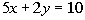Before we tackle finding the parallel and perpendicular slopes it really can help us out if we find the slope of the given line. Recall that when you are given the equation of a line that you can find the slope of it by writing it in the slope/intercept form,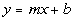, where m is the slope and b is the y-intercept of the line. Rewriting this equation in the slope/intercept form we get: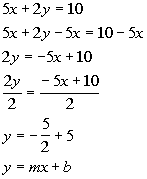*Inverse of add. 5x is sub. 5x *Inverse of mult. by 2 is div. by 2   *Written in slope/intercept form

 Lining up the form with the equation we got, can you see what the slope is? If you said -5/2, you are correct!!!   Slope of the parallel line: Since parallel lines have the same slope what do you think the slope of the parallel line is going to be? Pat yourself on the back if you said -5/2. Slope of the perpendicular line: Since the slopes of perpendicular lines are negative reciprocals of each other, what do you think the slope of the perpendicular line is?  Give yourself a high five if you said 2/5. Remember that you take the reciprocal which is -2/5 and then you negate it to get the 2/5 for your perpendicular slope.   The slope of the parallel line is -5/2 and the slope of the perpendicular line is 2/5.Answer/Discussion to 1bDo you remember what special type of line this equation is?  It is a horizontal line.  If you need a review on horizontal lines, feel free to go to Tutorial 27: Graphing Lines. What is the slope of a horizontal line?  If you said 0, you are right on.   Slope of the parallel line: Since parallel lines have the same slope, what do you think the slope of the parallel line is going to be? Pat yourself on the back if you said 0.   Slope of the perpendicular line: Since slopes of perpendicular lines are negative reciprocals of each other, what do you think the slope of the perpendicular line is?  This one is a little trickier.  Vertical lines and horizontal lines are perpendicular to each other.  The slope of the perpendicular line in this case would be the slope of a vertical line which would be undefined.    The slope of the parallel line is 0 and the slope of the perpendicular line is undefined.Answer/Discussion to 1cDo you remember what special type of line this equation is?  It is a vertical line.  If you need a review on vertical lines, feel free to go to Tutorial 27: Graphing Lines. What is the slope of a vertical line?  If you said undefined, you are right on.   Slope of the parallel line: Since parallel lines have the same slope, what do you think the slope of the parallel line is going to be? Pat yourself on the back if you said undefined.   Slope of the perpendicular line: Since slopes of perpendicular lines are negative reciprocals of each other, what do you think the slope of the perpendicular line is?  This one is a little trickier.  Vertical lines and horizontal lines are perpendicular to each other.  The slope of the perpendicular line in this case would be the slope of a horizontal line which would be 0.    The slope of the parallel line is undefined and the slope of the perpendicular line is 0.Answer/Discussion to 2a Passes through (-7, 2) and is parallel to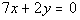.

 What are the two things we need to write an equation of a line???? If you said any point on the line and the slope, you are correct.   We have a point, however what about the slope?  Does this mean we can't work out the problem?  You are not going to get off that easily.  We need to do a little digging to get our slope. As mentioned above, parallel lines have the same slope.  So, if we know the slope of the line parallel to our line, we have it made.   Let's find the slope of the given line: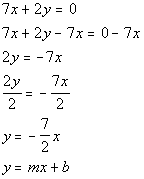*Inverse of add. 7x is sub. 7x   *Inverse of mult. by 2 is div. by 2 *Slope/intercept form of the line

 Now keep in mind that this is not the equation of our line but of the line parallel to our line.  We needed to write it this way so we could get the slope.  And it looks like the slope is -7/2.  Since our line is parallel to a line that has a slope of -7/2, our line also has a slope of -7/2.  OK, now we have our slope, which is -7/2.  Now it is just like problems in Tutorial 26: Equations of Lines, we put the slope and one point into the point/slope equation. Point/Slope Form: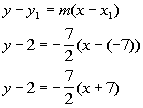*Point/slope form of the line

 Make sure that you are careful when one of your values is negative and you have to subtract it as we did in line 2.  x - (-7)  is not the same as x - 7.

 Next, we want to write it in the Slope/Intercept Form, which basically means we need to solve for y: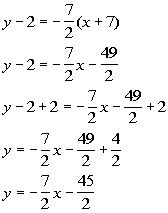*Dist. the -7/2 through (  ) *Inverse of sub. 2 is add. 2       *Slope/intercept form of the line

 The equation of the line that passes through (-7, 2) and is parallel tois  y - 2 = -7/2(x + 7)   OR   y = -7/2x - 45/2.Answer/Discussion to 2b Passes through (3, -1) and is perpendicular to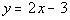.

 What are the two things we need to write an equation of a line???? If you said any point on the line and the slope, you are correct. We have a point, however what about the slope?  Does this mean we can't work out the problem?  You are not going to get off that easily.  We need to do a little digging to get our slope. As mentioned above, the slopes of perpendicular lines are negative reciprocals of each other.  So, if we know the slope of the line perpendicular to our line, we have it made.   Let's find the slope of the given line: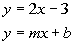*Slope/intercept form of the line

 Now keep in mind that this is not the equation of our line but of the line parallel to our line.  We needed to write it this way so we could get the slope.  And it looks like the slope is 2.  Since our line is perpendicular to a line that has a slope of 2, our line has a slope of -1/2 (the negative reciprocal of 2). OK, now we have our slope, which is -1/2.  Now it is just like problems in Tutorial 26: Equations of Lines, we put the slope and one point into the point/slope equation. Point/Slope Form: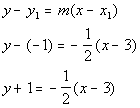*Point/slope form of the line

 Make sure that you are careful when one of your values is negative and you have to subtract it as we did in line 2.  y - (-1)  is not the same as y - 1.

 Next, we want to write it in the Slope/Intercept Form, which basically means we need to solve for y: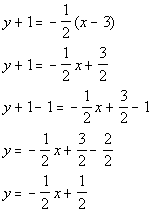*Dist. the -1/2 through (  )     *Inverse of add. 1 is sub. 1        *Slope/intercept form of the line

 The equation of the line that passes through (3, -1) and is perpendicular tois y + 1 = -1/2(x - 3)  OR   y = -1/2x + 1/2.

Last revised on Feb. 20, 2010 by Kim Seward.
All contents copyright (C) 2002 - 2010, WTAMU and Kim Seward. All rights reserved.